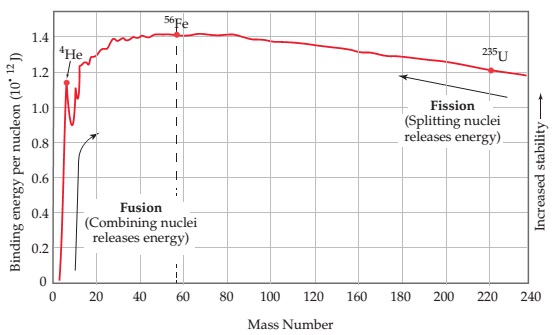# Problem: Which of the following nuclei is likely to have the largest mass defect per nucleon: 11B, 51V, 118Sn, 243Cm?

###### FREE Expert Solution

We’re being asked to determine which of the following nuclei is likely to have the largest mass defect per nucleon: 11B, 51V, 118Sn, 243Cm based on the plot of their binding energy.

To do so, we need to do the following steps,

Step 1: Based on the plot, determine the nucleon with the highest binding energy.
Step 2: Determine the nucleon with the largest mass defect (Δm) in kg, using the equation:

$\overline{){\mathbf{E}}{\mathbf{=}}{\mathbf{∆}}{\mathbf{m}}{{\mathbf{c}}}^{{\mathbf{2}}}}$

E = binding energy in J

Δm = mass defect in kg
c = speed of light = 3.0x108 m/s

Step 1: From the plot, we can see that the binding energy of each nucleon increases:

• from left to right  → as mass numbers increase during fusion
84% (13 ratings)###### Problem DetailsWhich of the following nuclei is likely to have the largest mass defect per nucleon: 11B, 51V, 118Sn, 243Cm?

Frequently Asked Questions

What scientific concept do you need to know in order to solve this problem?

Our tutors have indicated that to solve this problem you will need to apply the Mass Defect concept. If you need more Mass Defect practice, you can also practice Mass Defect practice problems.

What professor is this problem relevant for?

Based on our data, we think this problem is relevant for Professor Davis' class at UCF.